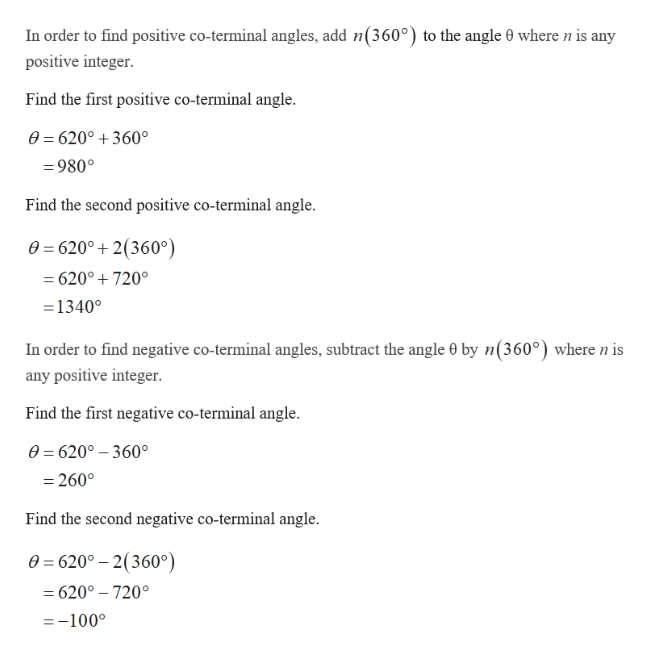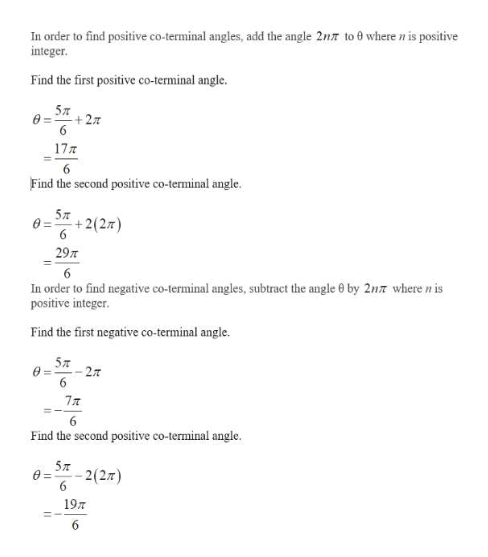# If the given angle is in standard position, find two positive coterminal angles and two negative co- terminal angles. a. 620 degreesb. 5pi/6c. - pi/4

Question
2 views

If the given angle is in standard position, find two positive coterminal angles and two negative co- terminal angles.

a. 620 degrees

b. 5pi/6

c. - pi/4

check_circle

star
star
star
star
star
1 Rating
Step 1

a) The angle θ=620֯ in standard position.help_outlineImage TranscriptioncloseIn order to find positive co-terminal angles, add n(360°) to the angle 0 wheren is any positive integer. Find the first positive co-terminal angle 0=620°+360° 980° Find the second positive co-terminal angle 0 6200 2(360° 620° 720° =1340° In order to find negative co-terminal angles, subtract the angle e by n(360°) where n is any positive integer. Find the first negative co-terminal angle. e 620° 360 260° Find the second negative co-terminal angle 0=620-2(360°) -620°-7200 -100° fullscreen
Step 2

b) The angle θ=5π/6 ...help_outlineImage TranscriptioncloseIn order to find positive co-terminal angles, add the angle 2nr to 0 where n is positive integer Find the first positive co-terminal angle. 5л +2 6 17T 6 Find the second positive co-terminal angle. 5л +2(27) 6 29m 6 In order to find negative co-terminal angles, subtract the angle 0 by 2n7 where n is positive integer. Find the first negative co-terminal angle. 5л 27 6 7T Find the second positive co-terminal angle. 5л 2(2) Ө- 6 19T 6 fullscreen

### Want to see the full answer?

See Solution

#### Want to see this answer and more?

Solutions are written by subject experts who are available 24/7. Questions are typically answered within 1 hour.*

See Solution
*Response times may vary by subject and question.
Tagged in

### Trigonometry